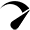Gravity is one of the fundamental forces in universe, and on our planet it affects every thing that has mass. Scientifically, gravity is the of force attraction between two masses and can be defined by the formula below:

F = G . (m1m2)/r

where: m1 and m2 are the masses of the two bodies and r is the distance between them.

G is the gravitational constant

For the case our planet, the major gravitational attraction comes from the earth itself. This is because the earth has the biggest mass (5.972 × 1024 kg) compared to the mass of any object on its surface. The gravitational attraction from the earth is a gravitational acceleration (9.81 m/s2) which moves objects towards the earth’s center. In the case of the engineering, gravity influences the loading and stability of the structural systems ,and in the following sections we are going look at why gravity is the biggest problem for engineers.

## Building Engineering

In building design, gravity contributes to most of the load that buildings are designed for. Buildings are normally designed to carry gravity loads such as the self –weight of the building and loads due to people using the building. Without gravity, buildings would carry much less load and even become more economical.

## Geotechnical Engineering

Geotechnical engineers focus on the analysis of the soil so as to determine whether it is stable and safe for a given purpose.  Geotechnical engineers have to solve problems such as slope stability and land slides which can be attributed to gravity. In the case of slope stability, gravity determines the weight of the soil within a slope and this consequently influences affects the stability of a given mass of soil as shown in the figure below.

## Hydraulic Engineering

Hydraulic engineering also deals with problems which are greatly influenced by gravity such as the control, storage and transportation of water. In order to store water in structures such as dam, the structures need to be design withstand the hydraulic pressure from the water and this pressure is due to the self weight of the water and it increases with depth as shown in the figure below. In the transportation of the water, engineers sometimes to pump water against gravity especially to points at a high altitude ,and this can be costly.

## Wind Engineering

Gravity is the root cause of the wind pressure and it influences the speed and direction of wind. Earth’s atmosphere is compressed by gravity resulting in air pressure ,and hence the presence of pressure gradients which cause wind. Therefore, engineers have their work cut out as they analyze wind movements and pressures so as to ensure that structures remain stable and safe when impacted which wind.

## Conclusion

Gravity is a fundamental part of our universe. Therefore, engineers have to find ways to deal with it. Whether it is the expansion our infrastructure on earth or the colonization of Mars, gravity is going to determine the nature of the structures we build and the principles we use.

## YOU MAY ALSO LIKE TO READ

#### FIVE MUST-HAVE GADGETS FOR ENGINEERSOptimized by Seraphinite Accelerator